### Home > CAAC > Chapter 6 > Lesson 6.1.3 > Problem6-31

6-31.
1. In an “If…then…“ statement, the “if” portion is called the hypothesis, while the “then” portion is called the conclusion. For example, in the statement “If x = 3, then x2 = 9,“ the hypothesis is “ x = 3” while the conclusion is “x2 = 9.”

Identify the hypothesis and conclusion of each of the following statements. Then decide if you think the statement is true or not. Justify your decision. Homework Help ✎

1. If −x = 8 then x = −8.

2. If 3x + y = −11, then 6x + 2y = −22.

3. If Tomas runs at a constant rate of 4 meters every five seconds, then he will run 50 meters in 1 minute.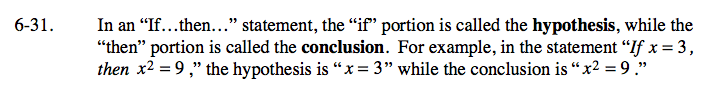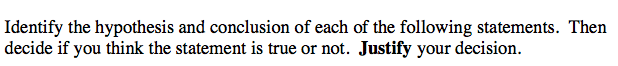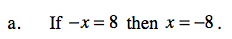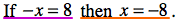Identify the hypothesis and the conclusion.

The statement is true.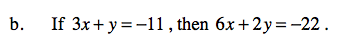Follow the steps in part (a).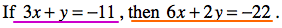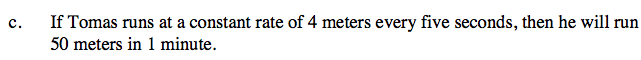Follow the steps in part (a).